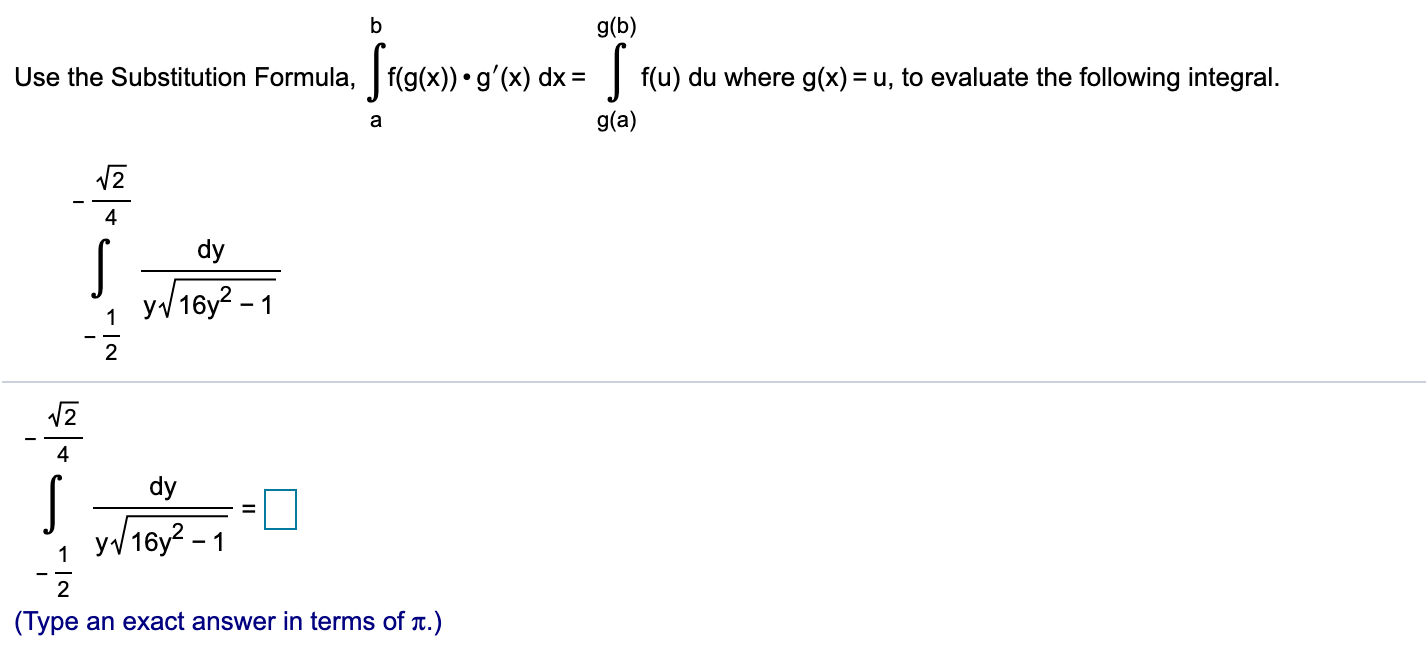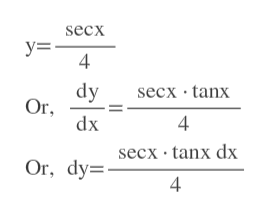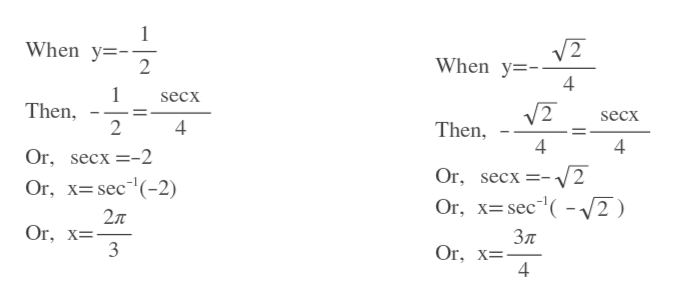# g(b)Use the Substitution Formula,f(g(x)). g'(x) dxf(u) du where g(x) = u, to evaluate the following integral.g(a)a4.dyy16y2-1224dyy16y2-112(Туреan exact answer in terms of t.)

Question
95 views

Use the Substitution​Formula,

Integral from a to b f left parenthesis g left parenthesis x right parenthesis right parenthesis times g prime left parenthesis x right parenthesis dx equals Integral from g left parenthesis a right parenthesis to g left parenthesis b right parenthesis f left parenthesis u right parenthesis du

where

​g(x)equals
​u,

to evaluate the following integral.help_outlineImage Transcriptioncloseg(b) Use the Substitution Formula,f(g(x)). g'(x) dx f(u) du where g(x) = u, to evaluate the following integral. g(a) a 4. dy y16y2- 1 2 2 4 dy y16y2-1 1 2 (Туре an exact answer in terms of t.) fullscreen
check_circle

Step 1

Let us assume y=(sec x)/4help_outlineImage Transcriptionclosesecx у3 4 dy Or dx secx tanx 4 secx tanx dx Or, dy fullscreen
Step 2

When y=-1/2, then x=2pi/3

When y=-...help_outlineImage TranscriptioncloseWhen y- 2 V2 When y 4 secx Then 2 V2 secx Then 4 4 4 Or, secx-2 Or, secx=- Or, х3 sec"(-2) Or, Xsec V2 27T Or, x- Зл Or, x= 4 fullscreen

### Want to see the full answer?

See Solution

#### Want to see this answer and more?

Solutions are written by subject experts who are available 24/7. Questions are typically answered within 1 hour.*

See Solution
*Response times may vary by subject and question.
Tagged in

### Calculus